# Confirmation that Average Power Consumption is within the Rated Power

2018.02.08

・In the case of continuous-pulse (switching) operation, the average power consumption is determined, and it is confirmed that allowable power dissipation is within the rated value.

・A judgment will in the end depend on whether Tj exceeds the absolute maximum rating.

In this chapter, we explain methods and procedures for determining whether or not a selected transistor is appropriate in actual operation.

This time, we explain ⑥”Confirmation that average power consumption is within the rated power” in the flowchart on the right. The discussion thus far has assumed switching operation, and so the selection in ⑤ is “continuous pulse”.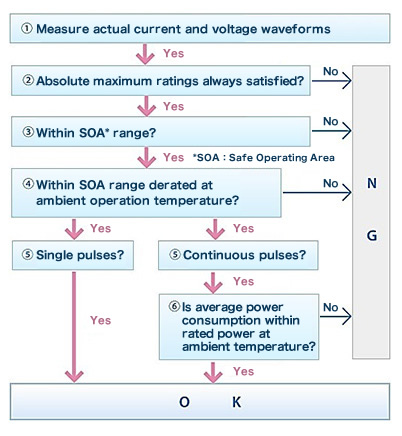## ⑥Confirmation that Average Power Consumption is within the Rated Power

In the previous section, we confirmed that even upon performing the necessary derating for the actual operating temperature conditions, operation was within the SOA range. This time, we determine the average power consumption for a case in which the operation is switching operation (continuous pulse), and check whether the operation is within the rated range. This is necessary because, as explained in ③, the SOA conditions are for “single pulse” operation, and so cannot be applied directly to a case of continuous pulse operation, such as switching operation. Hence as an additional check, we confirm that power consumption is within the rated range. In the case of switching operation, there is repetition of the transistor-on state in which current flows and power is consumed and the off-state in which there is no power consumption, and so it is necessary to determine the average power consumption.

## Method of Determining Average Power Consumption during Switching Operation

The average power consumption can be calculated by totaling the integrated values of the power, which is the product of the voltage and the current, for the interval of the transition from the transistor off-state to the on-state (t1-t2), the transistor on-time (t2-t3), the transition from on to off (t3-t4), and the off time (t4-t5), and dividing the result by the cycle (T). Please refer to the following diagram and equation.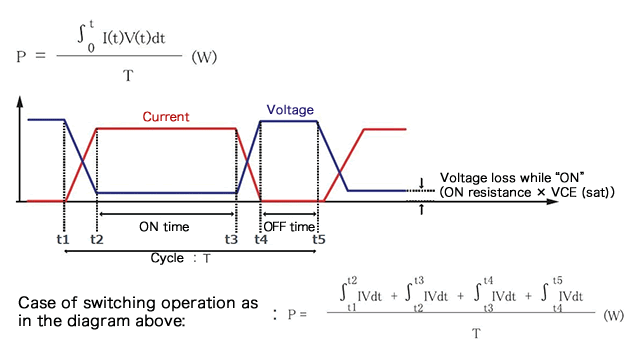The switching voltage/current waveforms which are measured and recorded in “①Measurement of the actual current and voltage waveforms” are used here.

Switching Waveforms of R6020ENZ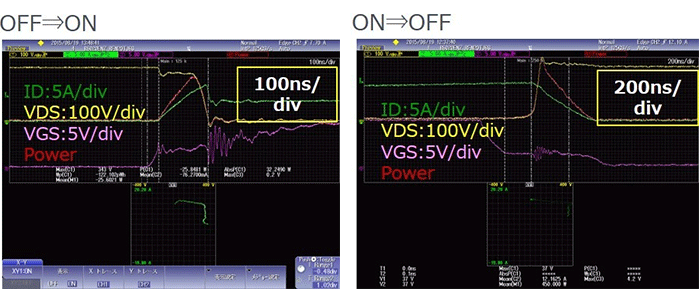Based on these waveforms, we perform calculations in accordance with the integral equation. This is for the example of a MOSFET, but if the device were a bipolar transistor, the same calculations could be performed, using the collector current IC and the collector-emitter voltage VCE.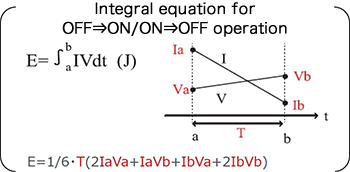If the average power consumption obtained through these calculations is within the maximum rated value for allowable power dissipation given on the data sheet, then we judge that losses do not pose a problem. Of course, it is necessary to determine whether the result is within the rated value for the operating temperature.

The following table gives the absolute maximum rated values for the R6020ENZ. The allowable power dissipation PD is 120 W, but we see that this is for the condition that TC = 25°C; under conditions other than this, the rated value cannot be applied as-is. In order to apply the correct value, we use a graph. This is the PD derating graph that was also used in “④Confirmation that operation is within the SOA derated at the ambient operating temperature”. In essence, this graph is used to judge whether or not use is possible by converting the power consumption into the equivalent Tj.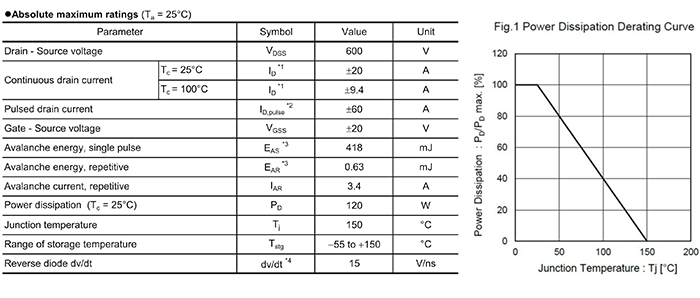In any case, Tj must be determined, and so ultimately it may be simpler to state that Tj must not exceed the absolute maximum rating under the applicable operating temperature conditions. We know the power consumption, and so if we determine the thermal resistance of the package, the calculation is easy. The following figures are also excerpted from the data sheet.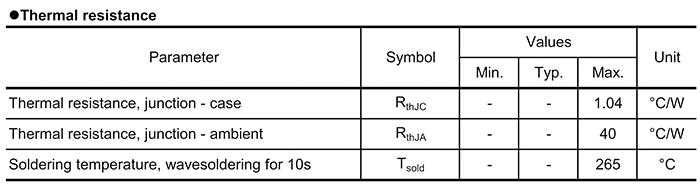The calculations are basically calculations to determine Tj, where Tj = power consumption × thermal resistance + operating temperature (maximum). There is no problem with using either Ta or Tc as the operating temperature, according to convenience.

There are a number of methods for confirming that the power consumption is within the rated value, but they are in the end equivalent to determining whether Tj is within the absolute maximum rated value. In the next section, we will explain calculation of Tj in somewhat greater detail.